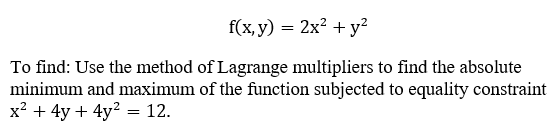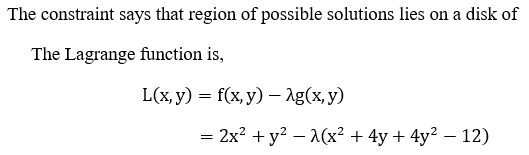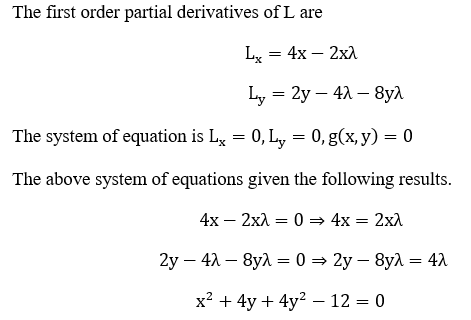# The function ​f(x,y)=2x2+y2 has an absolute maximum value and absolute minimum value subject to the constraint x2+4y+4y2 =12.Use Lagrange multipliers to find these values.The absolute maximum and absolute minimum.

Question
4 views
The function ​f(x,y)=2x2+y2 has an absolute maximum value and absolute minimum value subject to the constraint x2+4y+4y2 =12.
Use Lagrange multipliers to find these values.
The absolute maximum and absolute minimum.
check_circle

Given a functionNote:Calculation:...

### Want to see the full answer?

See Solution

#### Want to see this answer and more?

Solutions are written by subject experts who are available 24/7. Questions are typically answered within 1 hour.*

See Solution
*Response times may vary by subject and question.
Tagged in